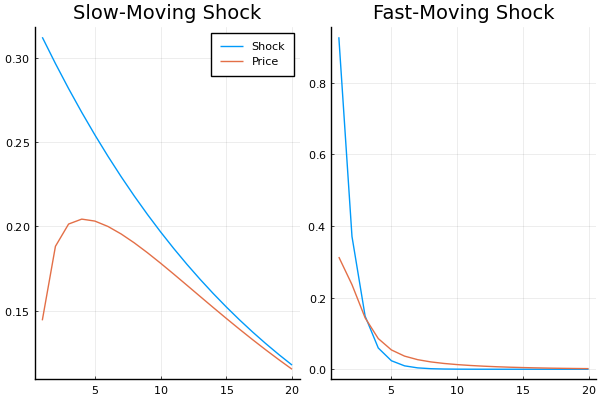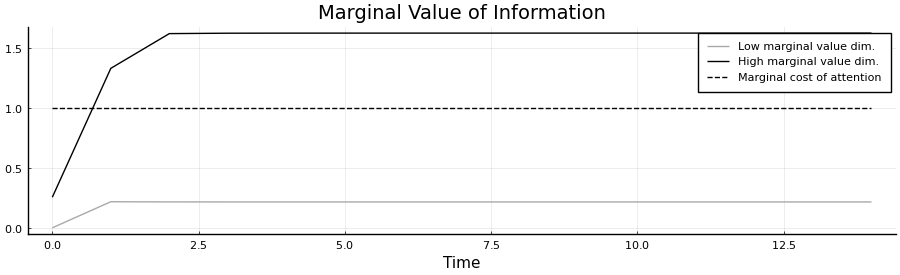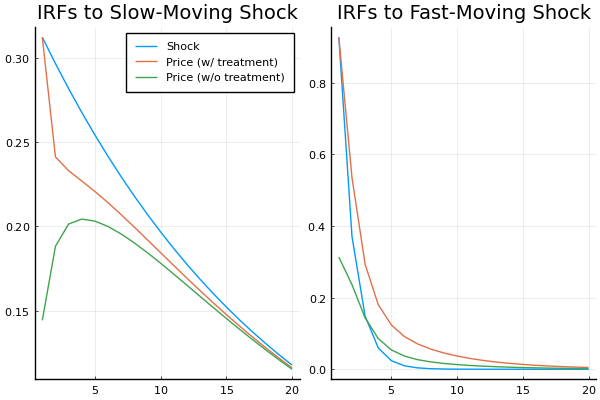DRIPs.jl: A Toolbox for Solving
Dynamic Rational Inattention Problems

Hassan Afrouzi          Choongryul Yang
Columbia U.          Federal Reserve Board

Motivation

• There is growing demand for departures from full information models:
• Households and firms make mistakes in perceiving available information
• Rational inattention offers an appealing alternative:

• A model that introduces a cost to information acquisition
• But has enough discipline to still generate robust predictions
• Dynamics are notoriously hard/slow to solve.

• Both state and choice variables are distributions with endogenous support.
• Very difficult to solve for continuous action/state sets.
• Easiest case: Linear Quadratic Gaussian (LQG) setting.

• State and choice variables reduce to $n$-dimensional variance-covariance matrices.

Dynamic Rational Inattention Problems (DRIPs)

\begin{align*} \min_{\{\vec{a}_t\}_{t\geq 0}} & \ \ \mathbb{E}\left[\sum_{t=0}^\infty \beta^t \bigg( (\vec{a}_t - \vec{x}_t'\mathbf{H})'(\vec{a}_t - \vec{x}_t'\mathbf{H}) - \omega \mathbb{I}(\vec{a}_t;\vec{x}_t|\vec{a}^{t-1}) \bigg) \lvert \vec{a}^{-1}\right] \\ s.t. \ \ & \ \ \vec{x}_t=\mathbf{A}\vec{x}_{t-1}+\mathbf{Q}\vec{u}_t,\quad \vec{u}_t\sim \mathcal{N}(\mathbf{0},\mathbf{I}^{k\times k}) \\ & \ \ \vec{a}^t=\vec{a}^{t-1} \cup a_t \end{align*}
• $\vec{a}_t\in\mathbb{R}^m$ is the vector of the agent's actions at time $t$.
• $\vec{x}_t\in\mathbb{R}^n$ is the vector of the shocks that the agent faces at time $t$.

Why is this hard to solve? Solution is not necessarily interior: \begin{align*} \mathbb{I}(\vec{a}^t;\vec{x}^t|\vec{a}^{t-1}) = \sum_{j=1}^n \mathbb{I}(\vec{a}_t;x_{t,j}|\vec{a}^{t-1},x_{t}^{j-1}) \\ \mathbb{I}(\vec{a}_t;x_{t,j}|\vec{a}^{t-1},x_{t}^{j-1}) \geq 0, \forall 1\leq j\leq n \end{align*}

Solution

• The solution to the dynamic rational inattention problem is a joint stochastic process between the actions and the states: $\{(\vec{a}_t,\vec{x}_t):t\geq 0\}$, with an initial belief $\vec{x}_0\sim N(\hat{x}_0,\mathbf{\Sigma}_{0|-1})$.

• We are also interested in the law of motion for the agent's belief about $\vec{x}_t$ under the optimal information structure $\hat{x}_t=\mathbb{E}_t[\vec{x}_t]$ where the expectation is taken conditional on the agent's time $t$ information.

• Theorem 2.2 and Proposition 2.3 in Afrouzi and Yang (2020) characterize this joint distribution as a function of a sequence $(\mathbf{K_t},\mathbf{Y_t},\mathbf{\Sigma}_{z,t})_{t=0}^\infty$ where

\begin{align*} \vec{a}_t &= \mathbf{H}'\hat{x}_t = \mathbf{H}'\mathbf{A}\hat{x}_{t-1}+\mathbf{Y}_t'(\vec{x}_t-\mathbf{A}\hat{x}_{t-1})+\vec{z}_t \\ \hat{x}_t &= \mathbf{A}\hat{x}_{t-1}+\mathbf{K}_t\mathbf{Y}_t'(\vec{x}_t-\mathbf{A}\hat{x}_{t-1})+\mathbf{K}_t\vec{z}_t,\quad \vec{z}\sim\mathcal{N}(0,\Sigma_{z,t}) \end{align*}

Solution

\begin{align*} \vec{a}_t &= \mathbf{H}'\hat{x}_t = \mathbf{H}'\mathbf{A}\hat{x}_{t-1}+\mathbf{Y}_t'(\vec{x}_t-\mathbf{A}\hat{x}_{t-1})+\vec{z}_t \\ \hat{x}_t &= \mathbf{A}\hat{x}_{t-1}+\mathbf{K}_t\mathbf{Y}_t'(\vec{x}_t-\mathbf{A}\hat{x}_{t-1})+\mathbf{K}_t\vec{z}_t,\quad \vec{z}\sim\mathcal{N}(0,\Sigma_{z,t}) \end{align*}
• $\mathbf{K}_t\in\mathbb{R}^{n\times m}$ is the Kalman-gain matrix of the agent in under optimal information acquisition

• $\mathbf{Y}_t\in\mathbb{R}^{n\times m}$ maps the state to the agent's action under rational inattetion

• $\mathbf{\Sigma}_{z,t}\in\mathbb{R}^{m\times m}$ is the variance-covariance matrix of the agent's rational inattention error

• DRIPs.jl; Julia package with four functions to remember:

• p = Drip(ω,β,A,Q,H): define and solve for the steady state of the problem.
• pt = Trip(p,S): solve for the transition dynamics of p with initial condition S.
• irfs(pt): solve for the impulse responses of actions and beliefs.
• simulate(p): simulate a path for actions and beliefs.

Installing and using DRIPs

In :
using Pkg; Pkg.add("DRIPs");
using DRIPs;

   Updating registry at ~/.julia/registries/General
Resolving package versions...
No Changes to ~/Dropbox/Work/Papers/Dynamic_Inattention/GitHub/Package/Slides/Project.toml
No Changes to ~/Dropbox/Work/Papers/Dynamic_Inattention/GitHub/Package/Slides/Manifest.toml


Sims (2011)

A tracking problem with two AR(1) shocks with different persistence: $a_t = \mathbb{E}_t[x_{1,t} + x_{2,t}]$. So $\vec{x}_t=(x_{1,t},x_{2,t})$.

The problem in Sims (2011), as it appears on page 21, with slight change of notation, \begin{aligned} & \min_{\{\Sigma_{t|t}\succeq 0\}_{t\geq 0}} \mathbb{E}_0\left[\sum_{t=0}^\infty \beta^t \left(tr(\Sigma_{t|t}\mathbf{H}\mathbf{H}')+\omega\log\left(\frac{|\Sigma_{t|t-1}|}{|\Sigma_{t|t}|}\right)\right)\right] \\ s.t.\quad & \Sigma_{t+1|t}=\mathbf{A}\Sigma_{t|t}\mathbf{A}'+\mathbf{Q}\mathbf{Q}'\\ & \Sigma_{t|t-1}-\Sigma_{t|t} \text{ positive semi-definite} \end{aligned}

\begin{aligned} \mathbf{H} = \left[\begin{array}{c} 1 \\ 1\end{array}\right], \quad \mathbf{A} = \left[\begin{array}{cc} 0.95 & 0\\ 0 & 0.4\\ \end{array}\right], \quad \mathbf{Q} = \left[\begin{array}{cc} \sqrt{0.0975} & 0\\ 0 & \sqrt{0.86}\\ \end{array}\right] \end{aligned}

Initialization

Set parameters:

In :
β = 0.9;
ω = 1.0;
A = [0.95 0.0; 0.0 0.4];
Q = [√0.0975 0.0; 0.0 √0.86];
H = [1.0; 1.0];


Include the package and define the problem:

In :
p = Drip(ω,β,A,Q,H)

Out:
Drip(1.0, 0.9, [0.95 0.0; 0.0 0.4], [0.3122498999199199 0.0; 0.0 0.9273618495495703], [1.0; 1.0], DRIPs.SteadyState([0.36299434366706423; 0.6370056563329357], [0.46227732429053797; 0.3378569310285822], [0.2928773543161892], [0.3595107310556166 -0.1772002380604728; -0.1772002380604728 0.7946194124703002], [0.421958434777694 -0.06733609046297966; -0.06733609046297966 0.987139105995248], [2.1686834434602282 1.242549121301687; 1.242549121301687 1.102665525912237], 9.145222436557738e-5))

What is in p?

p is of type Drip:

In :
@doc p

Out:

No documentation found.

p is of type Drip.

# Summary¶

struct Drip <: Any

# Fields¶

ω  :: Float64
β  :: Float64
A  :: Array{Float64,2}
Q  :: Array{Float64,2}
H  :: Array{Float64,2}
ss :: DRIPs.SteadyState

What is in p?

p.ss stores the solution to the steady-state information structure:

In :
ss = p.ss; @doc ss

Out:

No documentation found.

ss is of type DRIPs.SteadyState.

# Summary¶

struct DRIPs.SteadyState <: Any

# Fields¶

K   :: Array{Float64,2}
Y   :: Array{Float64,2}
Σ_z :: Array{Float64,2}
Σ_p :: Array{Float64,2}
Σ_1 :: Array{Float64,2}
Ω   :: Array{Float64,2}
err :: Float64

What is in p?

Here are the optimal covariances and the loading of price on shocks:

In :
p.ss.Σ_1 # prior in steady-state

Out:
2×2 Array{Float64,2}:
0.421958   -0.0673361
-0.0673361   0.987139
In :
p.ss.Σ_p # posterior is steady-state

Out:
2×2 Array{Float64,2}:
0.359511  -0.1772
-0.1772     0.794619
In :
p.ss.Y # loading of price on shocks in steady-state

Out:
2×1 Array{Float64,2}:
0.46227732429053797
0.3378569310285822

Stability and Performance: Solution Times

Solve for random value of $\beta$ and $\omega$ and measure solution times:

In :
using BenchmarkTools;
bench = @benchmark Drip(ω,β,A,Q,H) setup = (ω = 2*rand(), β=rand())

Out:
BenchmarkTools.Trial:
memory estimate:  291.64 KiB
allocs estimate:  2354
--------------
minimum time:     125.048 μs (0.00% GC)
median time:      133.924 μs (0.00% GC)
mean time:        150.040 μs (10.08% GC)
maximum time:     2.574 ms (93.81% GC)
--------------
samples:          10000
evals/sample:     1

IRFs

Use the built-in irfs() function to retrieve impulse response functions of beliefs and actions to shocks.

In :
p = Drip(ω,β,A,Q,H);        # Solve for the steady state of the DRIP
irfs_bp = irfs(p,T = 20);


What does irfs() return?

In :
@doc DRIPs.Path # type of output for the irfs() function

Out:

# Summary¶

A Structure for the irfs/simulations of DRIPs

# Fields¶

T     : length of IRFs/simulation
x     : IRFs/simulation of the fundamental shocks
x_hat : IRFs/simulation of beliefs
a     : IRFs/simulation of actions

In particular, if n is the dimension of x, m is the dimension of a and k is the number of structural shocks, then

• x has dimension n*k*T where x(i,j,:) is the impulse response function of the i'th dimension of x to the j'th structural shock.
• x_hat has dimension n*k*T where x_hat(i,j,:) is the impulse response function of the agent's average belief about the i'th dimension of x to the j'th structural shock.
• a has dimension m*k*T where a(i,j,:) is the impulse response function of the i'th action to the j'th structural shock.

Plot IRFs

In :
using Plots, LaTeXStrings; pyplot(); T  = 20;
pl1 = plot(1:T, [irfs_bp.x[1,1,:], irfs_bp.a[1,1,:]],
title             = "Slow-Moving Shock",
label             = ["Shock" "Price"])
pl2 = plot(1:T, [irfs_bp.x[2,2,:], irfs_bp.a[1,2,:]],
title             = "Fast-Moving Shock", legend = :false)
pl = plot(pl1,pl2,layout = (1,2))

Out:Transition Dynamics of Attention

• Suppose the initial belief is different from the Steady State belief.
• How does this change information acqusition?
• Important for understanding policies or studies that do one time treatments.
• Examples: information provision, RCTs, etc.
• The built in Trip function solves for transition dynamics.
• For instance let us consider a case where the firm is at the steady state of the rational inattention problem at time 0, and it receives a one time treatment with a perfectly informative signal about its optimal price: $s_0 = \mathbf{H}'\vec{x}_0$.
• Every signal has two parts: a loading matrix ($H$ in the example above) and a noise variance (0 in the example above).

Transition Dynamics of Attention

• Define the signal withDRIPs.Signal structure:
In :
signal = DRIPs.Signal(H,0.0)

Out:
DRIPs.Signal([1.0; 1.0], [0.0])
• Solve the transition dynamics:
In :
p = Drip(ω,β,A,Q,H);        # Solve for the steady state of the DRIP
Tss     = 15;               # guess for time until convergence back to SS
pt      = Trip(p, signal; T = Tss); # Solve for transition dynamics with initial treatment signal


Transition Dynamics of Attention

pt is of type Trip and stores the transition dynamics of information structure:

In :
@doc pt

Out:

No documentation found.

pt is of type Trip.

# Summary¶

struct Trip <: Any

# Fields¶

p       :: Drip
T       :: Int32
Σ_1s    :: Array{Float64,3}
Σ_ps    :: Array{Float64,3}
Ωs      :: Array{Float64,3}
Ds      :: Array{Float64,2}
err     :: Float64
con_err :: Float64

Transition Dynamics of Attention

Measure performance and stability for different randomly drawn treatments:

In :
using LinearAlgebra
@benchmark Trip(p, signal; T = 30) setup = (signal = DRIPs.Signal(rand(2,1),rand(1,1)))

Out:
BenchmarkTools.Trial:
memory estimate:  477.14 KiB
allocs estimate:  5120
--------------
minimum time:     410.319 μs (0.00% GC)
median time:      434.014 μs (0.00% GC)
mean time:        493.831 μs (11.30% GC)
maximum time:     6.023 ms (88.72% GC)
--------------
samples:          10000
evals/sample:     1

Transition Dynamics of Attention

How does prior variance converge back to steady state?

In :
pt.Σ_1s[:,:,1]

Out:
2×2 Array{Float64,2}:
0.323281  -0.323281
-0.323281   0.323281
In :
pt.Σ_1s[:,:,2]

Out:
2×2 Array{Float64,2}:
0.389261  -0.122847
-0.122847   0.911725
In :
pt.Σ_1s[:,:,3]

Out:
2×2 Array{Float64,2}:
0.420356   -0.0685534
-0.0685534   0.989063
In :
pt.Σ_1s[:,:,end]

Out:
2×2 Array{Float64,2}:
0.421634  -0.067235
-0.067235   0.987124

Transition Dynamics of Attention: Value of Information

In :
pl = plot(0:Tss-1,[pt.Ds[1,1:Tss],pt.Ds[2,1:Tss],pt.p.ω*ones(Tss,1)],
label             = ["Low marginal value dim." "High marginal value dim." "Marginal cost of attention"],
size              = (900,275),
title             = "Marginal Value of Information",
xlabel            = "Time",
color             = [:darkgray :black :black],
line              = [:solid :solid :dash])

Out:Transition Dynamics of Attention: IRFs

In :
tirfs_bp = irfs(pt, T=20); # irfs on the transition path
p1 = plot(1:T, [irfs_bp.x[1,1,:], tirfs_bp.a[1,1,:], irfs_bp.a[1,1,:]],
title             = "IRFs to Slow-Moving Shock",
label             = ["Shock" "Price (w/ treatment)" "Price (w/o treatment)"])
p2 = plot(1:T, [tirfs_bp.x[2,2,:], tirfs_bp.a[1,2,:], irfs_bp.a[1,2,:]],
title             = "IRFs to Fast-Moving Shock", legend = :false)
p = plot(p1,p2,layout     = (1,2))

Out: